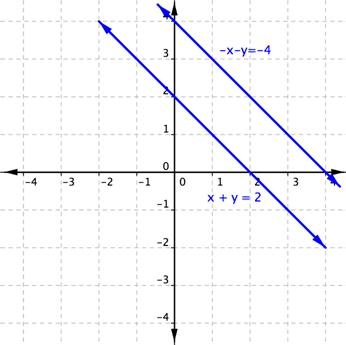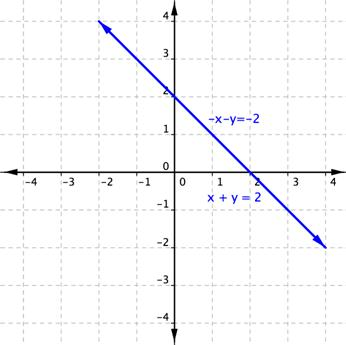## The Elimination Method Without Multiplication

### Learning Outcomes

• Use the elimination method without multiplication
• Express the solution of an inconsistent system of equations containing two variables
• Express the solution of a dependent system of equations containing two variables

The elimination method for solving systems of linear equations uses the addition property of equality. You can add the same value to each side of an equation to eliminate one of the variable terms.  In this method, you may or may not need to multiply the terms in one equation by a number first.  We will first look at examples where no multiplication is necessary to use the elimination method.  In the next section you will see examples using multiplication after you are familiar with the idea of the elimination method.

It is easier to show rather than tell with this method, so let’s dive right into some examples.

If you add the two equations,

$x–y=−6$ and $x+y=8$ together, watch what happens.

$\displaystyle \begin{array}{l}\,\,\,\,\,x-y=\,-6\\\underline{+\,x+y=\,\,\,8}\\\,2x+0\,=\,\,\,\,2\end{array}$

You have eliminated the y term, and this equation can be solved using the methods for solving equations with one variable.

Let’s see how this system is solved using the elimination method.

### Example

Use elimination to solve the system.

$\begin{array}{r}x–y=−6\\x+y=\,\,\,\,8\end{array}$

Let’s look an another example of solving by elimination that works out nicely.

### Example

Solve the given system of equations by elimination.

$\begin{array}{l}x+2y=-1\hfill \\ -x+y=3\hfill \end{array}$

Unfortunately not all systems work out this easily. How about a system like $2x+y=12$ and $−3x+y=2$. If you add these two equations together, no variables are eliminated.

$\displaystyle \begin{array}{l}\,\,\,\,2x+y=12\\\underline{-3x+y=\,\,\,2}\\-x+2y=14\end{array}$

But you want to eliminate a variable. So let’s add the opposite of one of the equations to the other equation. This means multiply every term in one of the equations by $-1$, so that the sign of every terms is opposite.

$\begin{array}{l}\,\,\,\,2x+\,\,y\,=12\rightarrow2x+y=12\rightarrow2x+y=12\\−3x+\,\,y\,=2\rightarrow−\left(−3x+y\right)=−(2)\rightarrow3x–y=−2\\\,\,\,\,5x+0y=10\end{array}$

You have eliminated the y variable, and the problem can now be solved.

The following video describes a similar problem where you can eliminate one variable by adding the two equations together.Caution!  When you add the opposite of one entire equation to another, make sure to change the sign of EVERY term on both sides of the equation. This is a very common mistake to make.

### Example

Use elimination to solve the system.

$\begin{array}{r}2x+y=12\\−3x+y=2\,\,\,\end{array}$

### Try It

The following are two more examples showing how to solve linear systems of equations using elimination.

### Example

Use elimination to solve the system.

$\begin{array}{r}−2x+3y=−1\\2x+5y=\,25\end{array}$

### Example

Use elimination to solve for $x$ and $y$.

$\begin{array}{r}4x+2y=14\\5x+2y=16\end{array}$

Go ahead and check this last example—substitute $(2, 3)$ into both equations. You get two true statements: $14=14$ and $16=16$!

Notice that you could have used the opposite of the first equation rather than the second equation and gotten the same result.

## Recognize systems that have no solution or an infinite number of solutions

Just as with the substitution method, the elimination method will sometimes eliminate both variables, and you end up with either a true statement or a false statement. Recall that a false statement means that there is no solution.

Let’s look at an example.

### Example

Solve for $x$ and $y$.

$\begin{array}{r}-x–y=-4\\x+y=2\,\,\,\,\end{array}$

Graphing these lines shows that they are parallel lines and as such do not share any point in common, verifying that there is no solution.### Try It

If both variables are eliminated and you are left with a true statement, this indicates that there are an infinite number of ordered pairs that satisfy both of the equations. In fact, the equations are the same line.

### Example

Solve for $x$ and $y$.

$\begin{array}{r}x+y=2\,\,\,\,\\-x−y=-2\end{array}$

Graphing these two equations will help to illustrate what is happening.### Try It

In the following video, a system of equations which has no solutions is solved using the method of elimination.

## Summary

Combining equations is a powerful tool for solving a system of equations. Adding or subtracting two equations in order to eliminate a common variable is called the elimination (or addition) method. Once one variable is eliminated, it becomes much easier to solve for the other one.

## Contribute!

Did you have an idea for improving this content? We’d love your input.